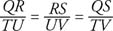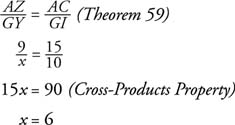## Proportional Parts of Similar Triangles

Theorem 59: If two triangles are similar, then the ratio of any two corresponding segments (such as altitudes, medians, or angle bisectors) equals the ratio of any two corresponding sides.

In Figure 1, suppose Δ QRS∼ Δ TUV.Figure 1 Corresponding segments of similar triangles.

Then,Then, according to Theorem 26Example 1: Use Figure 2 and the fact that Δ ABC∼ Δ GHI. to find x.Figure 2 Proportional parts of similar triangles.